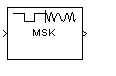# MSK Modulator Baseband

Modulate using differentially encoded minimum shift keying method

## Library

CPM, in Digital Baseband sublibrary of Modulation

•## Description

The MSK Modulator Baseband block modulates using the differentially encoded minimum shift keying method. The output is a baseband representation of the modulated signal.

This block accepts a scalar-valued or column vector input signal. For a column vector input signal, the width of the output equals the product of the number of symbols and the value for the Samples per symbol parameter.

### Pulse Shape Filtering

Differentially encoded minimum shift keying modulation uses pulse shaping to smooth the phase transitions of the modulated signal. The function q(t) is the phase response obtained from the frequency pulse, g(t), through this relation:$q\left(t\right)={\int }_{-\text{\hspace{0.17em}}\infty }^{t}g\left(t\right)dt$.

The specified frequency pulse shape corresponds to this rectangular pulse shape expression for g(t).

Pulse ShapeExpression
`Rectangular`$g\left(t\right)=\left\{\begin{array}{cc}\frac{1}{2LT},& 0\le t\le LT\\ 0& \text{otherwise}\end{array}$

• L is the main lobe pulse duration in symbol intervals.

• The duration of the pulse, LT, is the pulse length in symbol intervals.

### Integer-Valued Signals and Binary-Valued Signals

When you set the Input type parameter to `Integer`, then the block accepts values of 1 and -1.

When you set the Input type parameter to `Bit`, then the block accepts values of 0 and 1.

For information about the data types each block port supports, see the Supported Data Types table on this page.

### Single-Rate Processing

In single-rate processing mode, the input and output signals have the same port sample time. The block implicitly implements the rate change by making a size change at the output when compared to the input. In this mode, the input to the block can be multiple symbols.

• When you set Input type to `Integer`, the input can be a column vector, the length of which is the number of input symbols.

• When you set Input type to `Bit`, the input width must be an integer multiple of K, the number of bits per symbol.

The output width equals the product of the number of input symbols and the Samples per symbol parameter value.

### Multirate Processing

In multirate processing mode, the input and output signals have different port sample times. In this mode, the input to the block must be one symbol.

• When you set Input type to `Integer`, the input must be a scalar.

• When you set Input type to `Bit`, the input width must equal the number of bits per symbol.

The output sample time equals the symbol period divided by the Samples per symbol parameter value.

## Parameters

Input type

Indicates whether the input consists of bipolar or binary values.

The initial phase of the output waveform, measured in radians.

Samples per symbol

The number of output samples that the block produces for each integer or binary word in the input, which must be a positive integer. For all non-binary schemes, as defined by the pulse shapes, this value must be greater than 1.

Rate options

Select the rate processing option for the block.

• `Enforce single-rate processing` — When you select this option, the input and output signals have the same port sample time. The block implements the rate change by making a size change at the output when compared to the input. The output width equals the product of the number of symbols and the Samples per symbol parameter value.

• `Allow multirate processing` — When you select this option, the input and output signals have different port sample times. The output sample time equals the symbol period divided by the Samples per symbol parameter value.

Output data type

Specify the block output data type as `double` and `single`. By default, the block sets this to `double`.

## Supported Data Types

PortSupported Data Types

Input

• Double-precision floating point

• Boolean (when Input type set to `Bit`)

• 8-, 16-, and 32-bit signed integers (when Input type set to `Integer`)

Output

• Double-precision floating point

• Single-precision floating point

## Pair Block

MSK Demodulator Baseband

## References

 Anderson, John B., Tor Aulin, and Carl-Erik Sundberg, Digital Phase Modulation, New York, Plenum Press, 1986.

## Version History

Introduced before R2006a